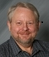## Sum of integer in table with .dbf formatTags:
Integer
Substring
I have table with .dbf format..inside only have 30 records which are 239,45,340,...etc. I wan to sum the integer like this..for example the first number..239=2+3+9=14..and the second number 4+5=9..then the output is 14,9,...until the 30th number..How would i do it? Help is desperately needed..I only know few tips..which are using for loop and substring...

Thanks. We'll let you know when a new response is added.

## Discuss This Question: 2 Replies

Thanks. We'll let you know when a new response is added.
•